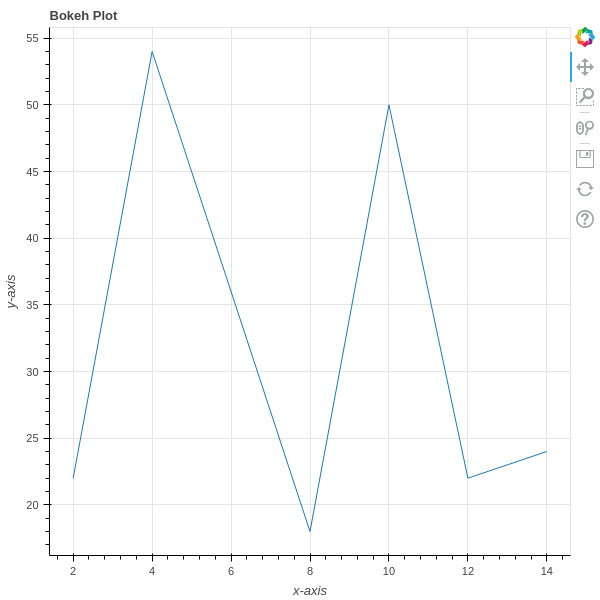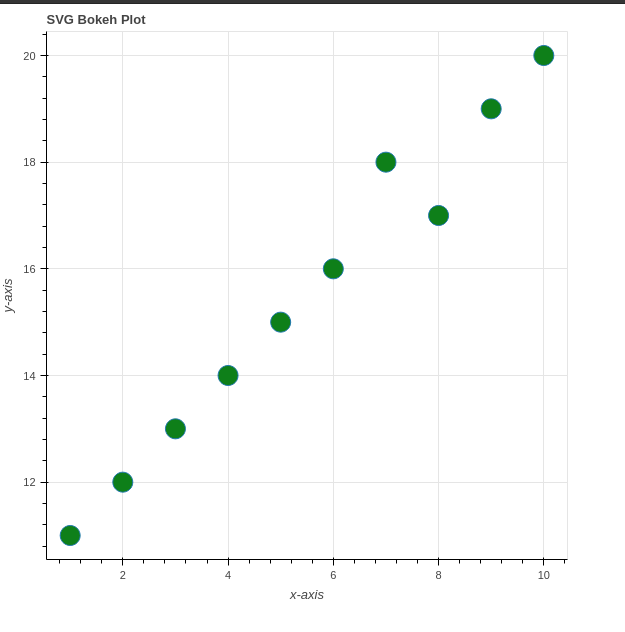Related Articles

# Exporting Bokeh Plots

• Last Updated : 28 Jul, 2021

Bokeh is an interactive data visualization library available for Python. Using Bokeh we can embed our plot in any HTML file. It internally uses HTML and JavaScript to render the plot in Web Browsers for representation. Under the hood, it converts the data source into a JSON file which is used as input for BokehJS (a JavaScript library) and renders the visualizations in modern browsers. In this article, we will see how we can export/save a Bokeh plot to local storage.

We will need the following dependencies to export the plots in bokeh:

• Selenium
• WebDriver

To install these two using conda, run the following command one after another:

```conda install selenium geckodriver -c conda-forge
conda install selenium python-chromedriver-binary -c conda-forge```

using pip:

`pip install selenium geckodriver firefox`

## Method 1: Using export_png() function to save plot as PNG

Using the export_png() function, we can export our plot as a PNG image directly from the Python code.

Syntax: export_png(obj, filename, width, height, webdriver)

Arguments:

• obj: obj can be any plot that we are going to export.
• filename: It is an optional argument, the default plot filename will be the python file name.
• width: It is an optional argument,  used to set the width of the exported plot layout obj, by default it will be ignored.
• height: It is an optional argument, used to set the height of the exported plot layout obj, by default it will be ignored.
• webdriver: It is an optional argument, used to set the default web driver instance to use to export the plot. A selenium web driver is by default if we don’t specify anything.

First, prepare the data to be visualized then call the figure() function to creates a plot with the default properties, such as its title and axes labels. Use the different varieties of renderers to create different varieties of plots. For example, to render a circle, we can use the circle() function instead of line() to render circles. Save the plot using export_png(plot_obj, filename) function and then display the resultant plot using show() function.

## Python3

 `# importing necessary libraries``from` `bokeh.plotting ``import` `figure``from` `bokeh.plotting ``import` `output_file``from` `bokeh.plotting ``import` `show``from` `bokeh.io ``import` `export_png`` ` `# dummy data``x ``=` `[``2``, ``4``, ``8``, ``10``, ``12``, ``14``]``y ``=` `[``22``, ``54``, ``18``, ``50``, ``22``, ``24``]`` ` `# set output to static HTML file``output_file(``"line.html"``)`` ` `# Adding plot``fig ``=` `figure(``    ``title``=``"Bokeh Plot"``,``    ``x_axis_label``=``'x-axis'``,``    ``y_axis_label``=``'y-axis'``,)`` ` `# add a line renderer to plot line``fig.line(x, y)`` ` `# saving the plot on disk``print``(``'Exporting bokeh_plot.png.....'``)``export_png(fig, filename ``=` `"bokeh_plot.png"``)`` ` `# displaying plot``show(fig)`

Output:

`Exporting bokeh_plot.png....`Exported as PNG

## Method 2: Using export_svg() function to save plot as SVG

Using the export_svg() function from bokeh.io, we can export our plot as an SVG image directly from the Python code.

Syntax: export_png(obj, filename, width, height, webdriver, timeout)

Arguments:

• obj: obj can be any plot that we are going to export.
• filename: It is an optional argument, the default plot filename will be the python file name.
• width: It is an optional argument,  used to set the width of the exported plot layout obj, by default it will be ignored.
• height: It is an optional argument, used to set the height of the exported plot layout obj, by default it will be ignored.
• webdriver: It is an optional argument, used to set the default web driver instance to use to export the plot. A selenium web driver is by default if we don’t specify anything.
• timeout: The maximum amount of time (in seconds) to wait for Bokeh to initialize. Its default value is 5s.

First, prepare the data to be visualized, then call the figure() function to creates a plot with the default properties, such as its title and axes labels. Use different varieties of renderers to create a different variety of plots and save the plot using export_svg(plot_obj, filename) function and then display the resultant plot using show() function.

## Python3

 `# importing necessary libraries``from` `bokeh.plotting ``import` `figure``from` `bokeh.plotting ``import` `output_file``from` `bokeh.plotting ``import` `show``from` `bokeh.io ``import` `export_svgs`` ` ` ` `# dummy data``x ``=` `[``1``, ``2``, ``3``, ``4``, ``5``, ``6``, ``7``, ``8``, ``9``, ``10``]``y ``=` `[``11``, ``12``, ``13``, ``14``, ``15``, ``16``, ``18``, ``17``, ``19``, ``20``]`` ` `output_file(``"circle_bokeh.html"``)`` ` `# Adding plot``fig ``=` `figure(``    ``title``=``"SVG Bokeh Plot"``,``    ``x_axis_label``=``'x-axis'``,``    ``y_axis_label``=``'y-axis'``,)`` ` `# add a circle renderer to plot``fig.circle(x, y, fill_color``=``"green"``, size``=``20``)`` ` `# saving the plot on disk``print``(``'Exporting circle_bokeh.svg.....'``)``fig.output_backend ``=` `"svg"``export_svgs(fig, filename ``=` `"circle_bokeh.svg"``)`` ` `# displaying plot``show(fig)`

Output:

`Exporting circle_bokeh.svg.....`Exported as SVG

Note: The exported plot will be saved in the folder where the python code file is presented.

Attention geek! Strengthen your foundations with the Python Programming Foundation Course and learn the basics.

To begin with, your interview preparations Enhance your Data Structures concepts with the Python DS Course. And to begin with your Machine Learning Journey, join the Machine Learning – Basic Level Course

My Personal Notes arrow_drop_up